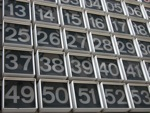1

## Last Digit Trick

Published on Sunday, January 13, 2013 in ,Quick, without using a calculator, what's the last (rightmost) digit of 11,467,51934,573?

Believe it or not, after reading and practicing the techniques in this post, you should be able to answer that quickly!

I'll start with the good news. When you're looking solely for the last digit of any exponential expression of the form xy, in which x and y are positive integers (whole numbers), it turns out that you can reduce x to a number from 0 to 9, reduce y to a number from 1 to 4, and get the same answer. If these two steps are done properly, you can easily work out the answer in your head.

PREREQUISITES: In order to perform this, you should be able to instantly identify every multiple of 4 less than 100, know the squares of every number from 0 to 9, and know the cubes of every number from 0 to 9. If you've practiced and perform the root extraction feat and the 2-digit squaring feat, you're probably more than ready to handle this mental math feat.

POSSIBLE SHORTCUT: If the rightmost digit of x is 0, 1, 5, or 6, then the answer's rightmost digit will be the same, regardless of the exponent. For example, 12376 to any whole positive power will end in a 6, because the number itself ends in a 6. Similarly, 8731 to any whole positive power will end in a 1, and so on. Because of this, you have a 40% chance of being able to give the answer without a single calculation!

ELIMINATE IRRELEVANT INFORMATION: If the number doesn't end in 0, 1, 5, or 6, you'll need to do a little more work. This first step can be done without thinking.

When given your xy expression, simply ignore all but the rightmost digit of x, and the two rightmost digits of y (due to the rule about divisblity by 4). The example problem given at the beginning of this post, 11,467,51934,573, reduces to the far simpler 973.

ELIMINATE MULTIPLES OF 4 IN Y: Once you get y down a number less than 100, as yourself whether y is a multiple of 4. If it is a multiple of 4, then you can simply think of the problem as being to the 4th power.

As an example, 239,478157,376 reduces to 876 in the first step. Since 76 is a multiple of 4, this second step reduces the problem to 84, which is a much more manageable problem.

If y isn't a multiple of 4, simply subtract the nearest multiple of 4 that is less than y. Returning to our reduced example of 973, we see that 73 is not a multiple of 4, so we subtract the nearest multiple of 4 less than 73, which is 72. 73 - 72 = 1, so the fearsome-looking 11,467,51934,573 will have the same last digit as the far simpler problem of 91.

WORK OUT THE LAST DIGIT OF THE REDUCED ANSWER: Once you've reduced x to a number from 0 to 9, and y to a number from 1 to 4, you should be able to get the last digit in your head.

If y reduces to 1, you're done! Any number to the first power it itself. 11,467,51934,573 reducing down to 91 means the rightmost digit will be 9. 62289,137 boils down to 21 (do you see why?), and so will be 2. This easy result will happen about 25% of the time.

If y reduces to 2, simply square the reduced x, and give its last digit. 50,34824,738 reduces down to 82, which is 64. The last digit of 64 is 4, so you give the answer as 4.

Similarly, if y reduces to 3, simply cube x and give the last digit of that answer. If the given problem reduces to say, 73, you should know that's 343, and so be able to give 3 as the last digit of the answer.

Taking a number to the 4th power may seem intimidating, but there's an easy way to handle this. For any problem x4, simply square x, and then square only the last digit of that answer. So, if you're confronted with, say, 84, do 8 squared, which is 64, and then take the rightmost digit of that answer, 4, and square it. 4 squared is 16, so you give the last digit, 6, as your answer. This is easier than trying to work out the full answer for 84, which is 4,096, yet you get the same last digit!

As you can see, even the toughest of exponential expressions can be made manageable with this technique. You can have the person giving you the problem verify your answer with Wolfram|Alpha, as in this example.

Also, the more you perform this, the more you'll acquaint yourself with the patterns of the various numbers. For example, 9 to an even power will end in 1, and 9 to any odd power will end in 9. 4 to any even power will end in 6, and 4 to any odd power will end in 4.

Play around with this routine, and you'll have a fun, easy, and impressive skill!

### 1 Response to Last Digit TrickJay

This is great. I love stunts like this. I also enjoyed the Moon Phase Estimation from a few weeks ago. In honor of Easter coming up, you should give Conway's method for calculating Easter(Feel free to delete that part if you were planning on it and want to keep it a secret). These stunts are one of the reasons I keep coming back to this site.

PS. If you're found any way to simplify Conway's formula's for Rosh Hashana and Pass Over so it can be done mentally, please let me know. --Jay